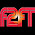## Tuesday, December 30, 2014

### 33 51 | The Death of Christine Cavanaugh

First, notice the CC, or 33 initials.
• Christine = 3+8+9+9+1+2+9+5+5 = 51
• Cavanaugh = 3+1+4+1+5+1+3+7+8 = 33
• Christine Cavanaugh = 84
• 12/22/14 = 12+22+14 = 48
Christine was born August 16, 1963.

#### 5 comments:

1.She made her career in 'voice over'.
Voice over: 22+15+9+3+5+15+22+5+18=114.
Voice over: 4+6+9+3+5+6+4+5+9=51.

2.3.This comment has been removed by the author.

4.It seems odd to me that CNN did not pick this story up until yesterday considering she died on Dec. 22? Maybe the family didn't tell anyone until then but I just find that odd.

51 is associated with conspiracy correct?

5.Yes that is correct Michael.
Area 51....
Conspiracy = 3+6+5+1+7+9+9+1+3+7 = 51